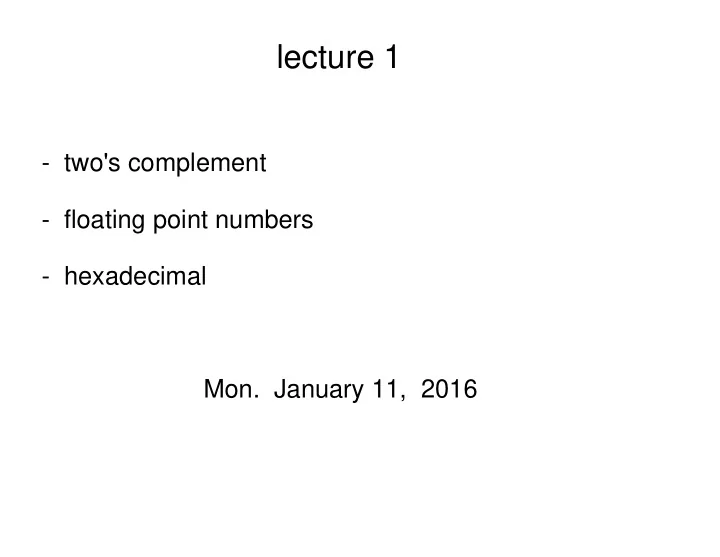# lecture 1 - two's complement - floating point numbers - - PowerPoint PPT Presentation

## lecture 1 - two's complement - floating point numbers - hexadecimal Mon. January 11, 2016 Car odometer (fixed number of digits) If you know what "modular arithmetic" is (MATH 240), then you recognize this: addition of integers

1. lecture 1 - two's complement - floating point numbers - hexadecimal Mon. January 11, 2016

2. Car odometer (fixed number of digits)

3. If you know what "modular arithmetic" is (MATH 240), then you recognize this: addition of integers mod 10^6.

4. Q: How to represent negative numbers in binary ? A: Given an 8 bit binary number m, define -m so that m + (-m) = 0.

5. Two's complement representation of integers Example: How to represent -26 ? Use a trick!

6. Another example: What is -0 ? m = 0 invert bits add 1 We have verified that -0 = 0.

7. What about m = 128 ? What is -128 ? m = 128 invert bits add 1 m = - 128 Thus, 128 is equivalent to -128.

8. binary "unsigned" "signed"

9. signed integers positive most significant bit negative

10. 8 bit integers (unsigned vs. signed)

11. n bits defines 2^n integers unsigned signed

12. Take n = 32. The largest signed integer is 2^31 - 1. 2 ^ 10 = 1024 ~ 10 ^ 3 = one thousand. 2 ^ 20 ~ 10 ^ 6 = one million 2 ^ 30 ~ 10 ^ 9 = one billion 2 ^ 31 ~ 2,000,000,000 = two billion

13. Java Example j = 4000000000; // 4 billion > 2^31 int This gives a compiler error. "The literal of type int is out of range." j = 2000000000; // 2 billion < 2^31 int System. out .println( 2 * j ); // This prints out -294967296. // To understand why these particular digits are printed, you // would need to convert 4000000000 to binary, which I don't // recommend.)

14. lecture 1 - two's complement - floating point numbers - hexadecimal Mon. January 11, 2016

15. Floating Point "decimal point" "binary point"

16. Convert from binary to decimal We must use both positive and negative powers of 2. Sum up the contributing 1 bits as on previous slide.

17. How to convert from decimal to binary ? To find the bits for the positive powers of 2, use the algorithm from last lecture ("repeated division") .

18. What about negative powers of 2 ? In general, note that multiplying by 2 shifts bits to the left (or shifts binary point to the right) Example:

19. Similarly....dividing by 2 and not ignoring remainder shifts bits to the right (or shifts binary point to the left)

20. For the negative powers of 2, use "repeated multiplication" convert decimal to binary

21. A more subtle example: First, find the bits for the positive powers of 2 using "repeated division" (last lecture).

22. Then find the bits for the negative powers of 2 using repeated multiplication.

23. Then find the bits for the negative powers of 2 using repeated multiplication. Note the summation is over bits bi from -5, -6, ..., - infinity.

24. We cannot get an exact representation using a finite number of bits for this example. Can we say anything more general about what happens ?

25. This will repeat over and over again.

26. When we convert a floating point decimal number with a finite number of digits into binary, we get: - a finite number of non-zero bits to left of binary point - an infinitely repeating sequence of bits to the right of the binary point Why ? [Note: sometimes the infinite number of repeating bits are all 0's, as in the case of 0.375 a few slides back.]

27. Recall previous example... Eventually, the three digits to the right of the decimal point will enter a cycle that repeats forever. This will produce a bit string that repeats forever.

28. Hexadecimal Writing down long strings of bits is awkward and error prone. Hexadecimal simplifies the representation.

29. Examples of hexadecimal 1) 0010 1111 1010 0011 2 f a 3 We write 0x2fa3 or 0X2FA3. 2) 101100 We write 0x2c (10 1100), not 0xb0 (1011 00)

30. See Exercises 1 Questions 1-7. Quiz 1 will be on Monday Jan 18. (15 minutes at start of class) Waiting list issues. (I will speak with admin.) OSD issues. They don't handle 15 min quizzes.

More recommend